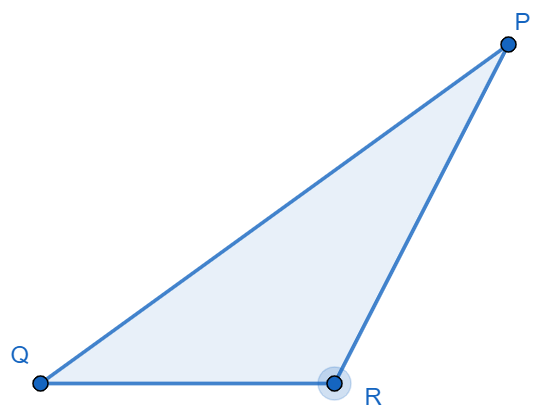Courses
Courses for Kids
Free study material
Offline Centres
MoreLast updated date: 07th Dec 2023
Total views: 282.3k
Views today: 7.82k

# In the adjoining figure: Name the vertex opposite to side $PQ$.Verified
Hint: In order to find the opposite vertex of the given side $PQ$ from the $\Delta PQR$, firstly, we must consider all the sides and the vertices. To find the opposite vertex of $PQ$, we must find such vertex from the $\Delta PQR$ such that it does not have any connection with the given particular side which simply means that it is not at all connected with the side $PQ$.
Now let us learn about the properties of a triangle. The angle-sum property of a triangle states that the sum of interior angles of a triangle is equal to ${{180}^{\circ }}$. The sum of lengths of any two sides of a triangle is greater than the third side of a triangle. There are six types of triangles. They are: equilateral triangle, isosceles triangle, scalene triangle, obtuse angled, right angled and acute angled triangle. The area of a triangle can be found by $\dfrac{1}{2}bh$ and the perimeter of a triangle can be found by sum of lengths of all three sides.
Now let us find the vertex that is opposite to the side $PQ$ from the triangle $\Delta PQR$.
From the figure, we can observe that the vertices $P$ and $Q$ are adjacent to the side $PQ$. In fact, we can say that the side $PQ$ is formed by those two vertices.
So, the only vertex that is not adjacent to the side $PQ$ is $R$.
$\therefore$ The vertex opposite to side $PQ$ is $R$.